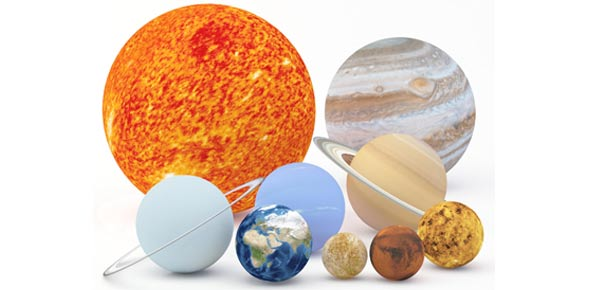# Astronomy Chapter Eleven

19 Questions | Total Attempts: 198SettingsRelated Topics
• 1.
Since all stars begin their lives with the same basic composition, what characteristic most determines how they will differ?
• A.

Location where they are formed

• B.

Time they are formed

• C.

Luminosity they are formed with

• D.

Mass they are formed with

• E.

Color they are formed with

• 2.
What are the standard units for luminosity?
• A.

Watts

• B.

Joules

• C.

Newtons

• D.

Kilograms

• E.

Watts per second

• 3.
A star's luminosity is the
• A.

Apparent brightness of the star in our sky.

• B.

Surface temperature of the star.

• C.

• D.

Total amount of light that the star will radiate over its entire lifetime.

• E.

Total amount of light that the star radiates each second.

• 4.
If the distance between us and a star is doubled, with everything else remaining the same,the luminosity
• A.

Is decreased by a factor of four, and the apparent brightness is decreased by a factor of four.

• B.

Is decreased by a factor of two, and the apparent brightness is decreased by a factor of two.

• C.

Remains the same, but the apparent brightness is decreased by a factor of two.

• D.

Remains the same, but the apparent brightness is decreased by a factor of four.

• E.

Is decreased by a factor of four, but the apparent brightness remains the same.

• 5.
Which of the following correctly states the luminosity-distance formula?
• A.

Luminosity = apparent brightness / 4π x (distance)^2

• B.

Apparent brightness = luminosity / 4π x (distance)^2

• C.

Apparent brightness = luminosity x 4π x (distance)^2

• D.

Distance = luminosity / 4π x (apparent brightness)^2

• 6.
Suppose you measure the parallax angle for a particular star to be 0.1 arcsecond. Thedistance to this star is
• A.

10 light-years.

• B.

10 parsecs.

• C.

0.1 light-year.

• D.

0.1 parsec.

• E.

Impossible to determine.

• 7.
The spectral sequence sorts stars according to
• A.

Mass.

• B.

Surface temperature.

• C.

Luminosity.

• D.

Core temperature.

• E.

• 8.
The spectral sequence in order of decreasing temperature is
• A.

OFBAGKM.

• B.

OBAGFKM.

• C.

OBAFGKM.

• D.

ABFGKMO.

• E.

BAGFKMO.

• 9.
Which of the following terms is given to a pair of stars that appear to change positions in the sky, indicating that they are orbiting one another?
• A.

Visual binary

• B.

Eclipsing binary

• C.

Spectroscopic binary

• D.

Double star

• E.

None of the above

• 10.
Which of the following best describes the axes of a Hertzsprung-Russell (H-R) diagram?
• A.

Surface temperature on the horizontal axis and luminosity on the vertical axis

• B.

Mass on the horizontal axis and luminosity on the vertical axis

• C.

Surface temperature on the horizontal axis and radius on the vertical axis

• D.

Mass on the horizontal axis and stellar age on the vertical axis

• E.

Interior temperature on the horizontal axis and mass on the vertical axis

• 11.
On a Hertzsprung-Russell diagram, where would we find stars that are cool and dim?
• A.

Upper right

• B.

Lower right

• C.

Upper left

• D.

Lower left

• 12.
On a Hertzsprung-Russell diagram, where would we find stars that are cool and luminous?
• A.

Upper right

• B.

Lower right

• C.

Upper left

• D.

Lower left

• 13.
On a Hertzsprung-Russell diagram, where would we find stars that have the largest radii?
• A.

Upper right

• B.

Lower right

• C.

Upper left

• D.

Lower left

• 14.
On a Hertzsprung-Russell diagram, where would we find red giant stars?
• A.

Upper right

• B.

Lower right

• C.

Upper left

• D.

Lower left

• 15.
On a Hertzsprung-Russell diagram, where would we find white dwarfs?
• A.

Upper right

• B.

Lower right

• C.

Upper left

• D.

Lower left

• 16.
Which of the following luminosity classes refers to stars on the main sequence?
• A.

I

• B.

II

• C.

III

• D.

IV

• E.

V

• 17.
Which of the following is true about low-mass stars compared to high-mass stars?
• A.

Low-mass stars are cooler and less luminous than high-mass stars.

• B.

Low-mass stars are hotter and more luminous than high-mass stars.

• C.

Low-mass stars are cooler but more luminous than high-mass stars.

• D.

Low-mass stars are hotter but less luminous than high-mass stars.

• E.

Low-mass stars have the same temperature and luminosity as high-mass stars.

• 18.
Which of the following statements about an open cluster is true?
• A.

All stars in the cluster are approximately the same color.

• B.

All stars in the cluster are approximately the same age.

• C.

All stars in the cluster have approximately the same mass.

• D.

All stars in the cluster will evolve similarly.

• E.

There is an approximately equal number of all types of stars in the cluster.

• 19.
Cluster ages can be determined from
• A.

Main sequence fitting.

• B.

Main sequence turnoff.

• C.

Pulsating variable stars.

• D.

Spectroscopic binaries.

• E.

Cluster ages can be determined from A) main sequence fitting. B) main sequence turnoff. C) pulsating variable stars. D) spectroscopic binaries. E) visual binaries.